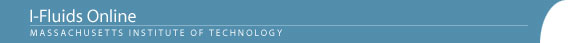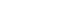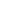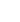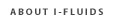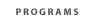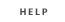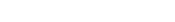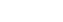2.25: Advanced Fluid Dynamics Section 1: Continuum viewpoint and the equation of motion Section 2: Static Fluids Section 3: Mass Conservation Section 4: Inviscid flow - differential approach Section 5: Control Volume Theorums Section 6: Navier-Stokes equation and viscous flow Section 7: Similarity and dimensional analysis Section 8: Boundary layers, separation and effect on drag/lift Section 9: Vorticity and circulation Section 10: Potential flows; lift, drag and thrust production Section 11: Surface tension and its effect on flows Section 12: Introduction to turbulence Back to 2.25 HomeSection 12: Basic Concepts in Turbulence Modeling12.1 Comments on laminar flow, its stability, and the transition to turbulent flow.
12.2 Features of turbulent flows (high Reynolds number, "randomness", three- dimensionality of fluctuations, intermittency near free boundaries, role of viscous dissipation, etc.).
12.3 The range of scales in turbulent motion; the Kolmogorov microscale. Limitations imposed by computer memory and speed on attempts to obtain exact numerical solutions (fluctuations and all!) of the Navier-Stokes equations for turbulent, high Reynolds number flows.
12.4 Statistical averages of random quantities in turbulent flow. Reynolds' equation for the mean flow; the Reynolds stress and the closure problem.
12.5 Prandtl's mixing length hypothesis: a simple "mean flow" (or "zero-equation") closure model.
12.6 Prandtl's closure hypothesis for free turbulent flows (jets, wakes). A rough back-of-the-envelope "solution" for a round turbulent jet.
12.7 The nature of wall-bounded turbulent flows: the outer and inner layers, the "universal law of the wall" for the inner layer; the logarithmic sublayer and the viscous sublayer. Derivation of the logarithmic mean-velocity profile from Prandtl's mixing length model.